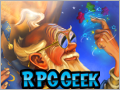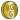SEARCH All RPG Item RPG System Series Setting Periodical Issue Article Podcast Podcast Ep. Designer Artist Production Staff Publisher Forums GeekLists Tags Wiki Users Adv. Search BrowseDatabase People & Companies Periodicals User Submitted Content Podcasts ForumsGeekListsBazaareBay MiscCreate HelpThe Hotness
Games|People|Company
BRAVE SIR KNIGHT Tavern Cryptogram
CLOSEDBRAVE SIR KNIGHT
Tavern Cryptogram

After solving the cryptogram, roll 1D10000. The closest to the original roll (4653) will win this challenge, and 50. The 2nd place will win 30. And 3rd place will win 20. All 3 will gain the title of Event Champion, and a commemorative MB. (If requested, you may need to send your solution to my geekmail address.) All who participate in this challenge will receive the microbadge:_ _ _ _ _ _ _ _ _ _ _ _ _ _ _ _ _ H _ _ _ H _
E V V Z I A I O H A X A P O I Y Z L I K U L I

_ _ _ _ _ _ _ _ _ H _ _ _ _ _ _ _ _ _ _ _ _ H _
K S M V I N K P D L U Z E V N I Y P K U S U L I

_ _ _ _ _ _ _ _ _ _ _ _ _ _ _ _ _ _ _ _ _ _ _
U E J I A K E K Y S A Y I A I Y R E A U P K P

_ _ _ _ _ _ _ _ _ _ _ _, _ _ _ H _ _ _ _
E C U I A R E A U P K P I E Q L U P R I

_ _ _ _ _ _ _ _ _ H _ _ _ _ _ _ _ _ _ _
A I R S J P K D U L I S V P J I O E K Y

_ _ _ _ _ _ _ _ H _ _ _ _ _ _ _ _. _ H _ _
X V E Q P K D U L I R P K E B E A Z L I K

_ H _ _ _ _ _ _ _ _ _ _ _ _ _ _ _ _ H
U L I B E A Z E O C P V V I Y Z P U L

_ _ _ _ _ _ _ _ _ _ _ _ _ H _ _ _ _ _ _ _
S V P J I O E K Y E V V U L I Y A P K N O

_ _ _ _ _ _ _ _, _ H _ _ _ _ _ H _ _ _ _ _ _ _ _
Q S K O H R I Y U L I N K P D L U O U E A U I Y

_ _ _ _ _ _ _. “_ _ _ _ _ _ _ _, _ _ _
U S V I E J I X E A Y S K R I O P A

_ _ _ _ H _.” _ _ _ _ _ H _ _ _ _ _ _ _ _, _ H _
N K P D L U O E P Y U L I M E A N I I X Z L S

_ _ _ _ _ _ _ _ _ _ _ _ _ _ _ H _ _ _ H _
Z E O X H W W V I Y S J I A Z L E U U L I

_ _ _ _ H _ H _ _ _ _ _ _. “_ H _ _ _ _ _ _ H _ _
N K P D L U L E Y Y S K I Z L E U Z E O U L E U

_ _ _ _ _ _ _ _?” ”_ _ _ H _ _ _.” _ _ _ _ _ H _
E V V E M S H U K S U L P K D O E P Y U L I

_ _ _ _ H _. “_ _ _ _ _ _ _ _ _ _ _ _ _
N K P D L U R F T H I I K O I K U R I

_ _ _ _ _ _ _ _ _ _ _ _ _ _ _ _ _ _.”
S H U C S A E B E A S C S V P J I O
• Edited Mon Oct 21, 2013 4:16 am
• Posted Sun Oct 6, 2013 3:07 pm
• [+] Dice rolls
• [+]
• 442306. XendoBreckett
• 1D10000 =
• (4653) =
• 4653
• Sun Oct 6, 2013 3:20 pm
• [+]
• 449299. kuni2
• d10000 =
• (2829) =
• 2829
• Fri Oct 18, 2013 6:06 pm
• [+]
• 449311. francisthe3rd
• d10000 =
• (2627) =
• 2627
• Fri Oct 18, 2013 6:32 pm
• [+]
• 449463. MichMedia
• 1D10000 =
• (485) =
• 485
• Sat Oct 19, 2013 1:59 am
• [+]
• 449492. Legolas_Katarn
• 1D10000 =
• (2848) =
• 2848
• Sat Oct 19, 2013 3:33 am
• [+]
• 449497. Crackerjack
• 1d10000 =
• (6492) =
• 6492
• Sat Oct 19, 2013 3:47 am
• [+]
• 449498. turtilian
• 1D10000 =
• (3699) =
• 3699
• Sat Oct 19, 2013 4:04 am
• [+]
• 449516. Astinex
• 1D10000 =
• (2038) =
• 2038
• Sat Oct 19, 2013 5:24 am
• [+]
• 449565. cornjerker
• d10000 =
• (8537) =
• 8537
• Sat Oct 19, 2013 10:41 am
• [+]
• 449573. susuris
• 1D10000 =
• (2214) =
• 2214
• Sat Oct 19, 2013 11:15 am
• [+]
• 449676. Paolo Robino
• 1d10000 =
• (6211) =
• 6211
• Sat Oct 19, 2013 5:38 pm
• [+]
• 449697. cafeine
• 1D10000 =
• (1315) =
• 1315
• Sat Oct 19, 2013 7:13 pm
• [+]
• 449727. Stelio
• 1D10000 =
• (6640) =
• 6640
• Sat Oct 19, 2013 10:19 pm
• [+]
• 449739. nyriv
• 1d10000 =
• (9167) =
• 9167
• Sat Oct 19, 2013 11:43 pm
• [+]
• d10000 =
• (3432) =
• 3432
• Sat Oct 19, 2013 11:49 pm
• [+]
• 449842. XendoBreckett
• 1D10000 =
• (3253) =
• 3253
• Sun Oct 20, 2013 2:35 am
• [+]
• 449845. Dutys_Fist
• 1d10000 =
• (6755) =
• 6755
• Sun Oct 20, 2013 2:55 am
• [+]
• 449867. bulldog93
• 1D10000 =
• (2497) =
• 2497
• Sun Oct 20, 2013 6:32 am
• [+]
• 449878. pikkusiili2000
• 1d10000 =
• (1935) =
• 1935
• Sun Oct 20, 2013 7:25 am
• [+]
• 449903. Jowolf
• 1d10000 =
• (2353) =
• 2353
• Jowolf
• Sun Oct 20, 2013 8:55 am
• [+]
• 449921. David Goldfarb
• 1d10000 =
• (9276) =
• 9276
• Sun Oct 20, 2013 11:53 am
• [+]
• 450044. jasri
• 1D10000 =
• (6953) =
• 6953
• Sun Oct 20, 2013 6:17 pm
• [+]
• 450107. sdonohue
• 1D10000 =
• (6157) =
• 6157
• Sun Oct 20, 2013 9:32 pm
• [+]
• 450183. leemc13
• d1000 =
• (313) =
• 313
• Mon Oct 21, 2013 4:38 am
• [+]
• 450184. leemc13
• d10000 =
• (4582) =
• 4582
• corrected my previous roll
• Mon Oct 21, 2013 4:38 am
 Front Page | Welcome | Contact | Privacy Policy | Terms of Service | Advertise | Support BGG | FeedsGeekdo, BoardGameGeek, the Geekdo logo, and the BoardGameGeek logo are trademarks of BoardGameGeek, LLC.